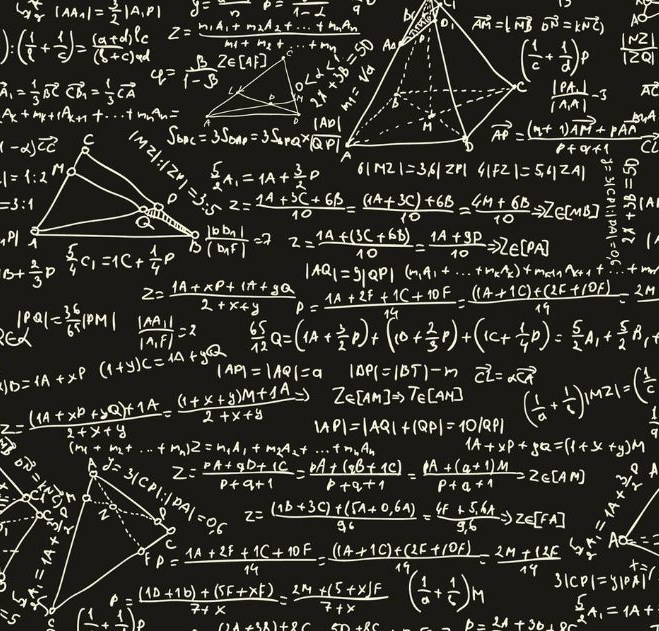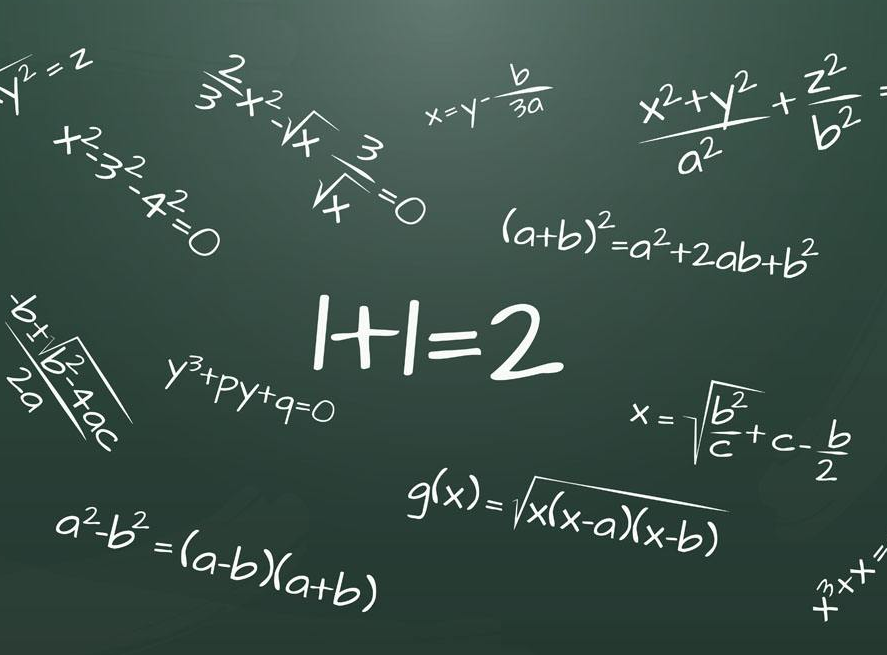# 数学作业代写案例：Power Flow Studies Using MATLAB

本文是数学专业的留学生作业代写范例，题目是“Power Flow Studies Using MATLAB(基于MATLAB的潮流研究)”报告的目的是显示牛顿-拉夫森和高斯-塞德尔方法之间的差异，通过使用它们来分析潮流系统。在双母线系统中，采用松弛母线和PQ母线两种收敛容限。案例研究1比较了电压容限收敛的两种方法的迭代过程，案例研究2比较了功率失配收敛的两种方法的迭代过程。研究的重点是结果和收敛速度。虽然这两种方法都收敛于相同的值，但Newton-Raphson是指数收敛的，而Gauss-Seidel是线性收敛的，只需大约一半的迭代就可以得到预期的结果。Executive Summary执行概要

The report aims to show the differences between Newton-Raphson and Gauss-Seidel methods by using them to analyse a power flow system. During the study in a two-bus system, with a slack bus and PQ bus, two convergence tolerances were used. The case study 1 compares the iterative process of the two methods for a voltage tolerance convergence and case study 2 compares the iterative process of the two methods for a power mismatch convergence. The main points that investigated are the results and the speed of convergence. Although, both methods converge to the same values, Newton-Raphson converges exponentially and compared to Gauss-Seidel which converges linearly, needs only around half iterations to return the expected result.

Executive Summary

Introduction

Background Theory

Newton-Raphson Method

Gauss-Seidel Method

Power Flow Study Results and Analysis

Case Study 1

Case Study 2

Analysis of the results and convergence characteristics acquired by the Newton-Raphson and Gauss-Seidel methods

Conclusions

References

Introduction介绍

A load or power flow analysis is a computational procedure required to determine the sinusoidal steady state of the entire power system. The mathematical solution includes a system of nonlinear algebraic equations, which during an iterative process, the values converge to only one solution. The outcome of the power flow analysis is the acquisition of the voltage magnitudes and angles at each bus. Once the voltage magnitudes and angles at each bus have been acquired using power flow analysis, the real and reactive power at each branch of the power system can be computed. Furthermore, losses in particular lines can be computed by investigating the difference between the power flow in the sending and receiving ends and by determining over and under load conditions, the appropriate solution is taken into consideration. In the power industry, load flow analysis is used on a daily basis as it is essential in determining the fault-free, stable and economical operation of existing power systems, as well as for future expansion of power systems.

负荷或潮流分析是一种计算过程，需要确定整个电力系统的正弦稳态。数学解包括一个非线性代数方程组，在迭代过程中，其值收敛到只有一个解。潮流分析的结果是采集每个母线的电压大小和角度。一旦通过潮流分析获得了各母线的电压大小和角度，就可以计算出电力系统各支路的实功率和无功功率。此外，通过研究发送端和接收端潮流的差异，并确定负载过负荷和负载下的情况，可以计算出特定线路的损耗，并考虑适当的解决方案。在电力工业中，潮流分析每天都在使用，因为它对确定现有电力系统的无故障、稳定和经济运行至关重要，也对电力系统的未来扩展至关重要。

A two-bus system is given in Figure 1 below.

Figure 1: Two-bus System

The power system consists of a slack bus, which is illustrated as bus 1 and a load bus, which is illustrated as bus 2.

Bus 1 is a slack bus (or swing, or reference) and its main role is to be the phase angle reference of the system. It also balances the active power |P| and reactive power |Q| in a system by injection in circumstances when is required. It is a reference bus for which the voltage magnitude and angle are input data (V1∠δ1)

and the active power |P| and reactive power |Q| are the unknown quantities need to be calculated by the power flow analysis. Usually the V1∠δ1

is 1.0∠0°

, however in this assignment should be considered as 1.06∠0°

Bus 2 is a load (P-Q) bus which absorbs power from the system. The active power |P| and reactive power |Q| are quantified and voltage magnitude |V| and angle |δ| need to be computed.

Background Theory背景理论

Newton-Raphson Method

The Newton-Raphson method is a powerful method for maximising an objective function by using quadratic convergence of approximations .

牛顿迭代法

Newton-Raphson方法是一种利用二次收敛逼近来最大化目标函数的有效方法。

A number of nonlinear algebraic equations is given in matrix format

fx=f1xf2x…=y                  (1.1)

where x and y are N vectors and fx

is an N vector of functions. The aim is to solve for x, accounting that fx

and y are given. By rewriting eq. 1.1

y–fx=0                        (1.2)

Then, by adding Dx to both of the eq. 1.2

y–fx+Dx=Dx           (1.3)

, where D is a square NxN invertible matrix. Then, multiplying by D–1

in both parts

x+D–1y–fx=x             (1.4)

The values of x on the left side are used to calculate the next values of x on the right side, so that

xi+1=xi+D–1y–fxi          (1.5)

For non-linear equations, it is necessary that matrix D must be specified. One of the methods of specifying matrix D called the Newton-Raphson method.

The Newton-Raphson method is based on the Taylor series expansion of   fx

.

y=fx0+dfdx|x=x0x–x0…          (1.6)

By neglecting the higher order terms in eq 1.6

x=x0+[dfdx|x=x0]–1 y–fx0             (1.7)

During the Newton Raphson iteration process, x0

is been replaced by the old value x and x by the new value  xi+1.

To continue to the next step, the N×N

Jacobian matrix is used.

Ji=dfdx|x=x(i)=∂f1∂x1∂f1∂x2…∂f1∂xN∂f2∂x1∂f2∂x2…∂f2∂xN…………∂fN∂x1∂fN∂x2…∂fN∂xN           (1.8)

where the elements inside the Jacobian matrix are partial derivatives. Therefore, by substituting the Jacobian matrix into eq. 1.7.

xi+1=xi+J–1(i) y–fxi

(1.9)

Instead of computing the inverse of the Jacobian matrix J–1

, it can be rewritten as :

JiΔxi= Δyi                (1.10)

, where       Δxi=xi+1–xi         1.11    and     Δyi= y–fxi         (1.12)

The Newton-Raphson method converges to the result in each iteration and depending on the convergence tolerance we can select the precision needed. The steps below show the process during each iteration.

Step 1   By using the eq. 1.12. we compute Δyi

Step 2  By using the eq. 1.8. we compute Ji

Step 3 By using Gauss elimination and back substitution, we solve the eq. 1.10 in order to find Δxi

Step 4 Finally we compute xi+1

from eq. 1.11. Compare xi+1

with xi

to find the convergence tolerance to determine the precision of the result. 

Power Mismatch

The equation for complex power expressed as Si

:

Si=Pi+jQi=ViIi*            (1.13)

From equation 1.13. the current injection into any bus I can be expressed as:

Ii=∑k=1nYikVk               (1.14)

where, Yik

terms are admittance matrix elements. Then, we substitute eq. 1.14 into eq. 1.13:

Si=Vi∑k=1nYikVk*              (1.15)

where, Vk is a phasor, with magnitude and angle Vi=|Vi|∠θi

. Moreover, the admittance matrix is complex with real and imaginary parts Yik=Gik+jBik

. Then, by rewriting eq. X as

Si=Vi∑k=1nYik*Vk*=|Vi|∠θi∑k=1n(Gik+jBik)*|Vk∠θk*            (1.16)

=|Vi|∠θi∑k=1n(Gik–jBik)(|Vk∠–θk              (1.17)

=∑k=1n|Vi|∠θi(|Vk|∠–θk)(Gik–jBik)        (1.18)

=∑k=1n(|Vi||Vk|∠θi–θk)(Gik–jBik)        (1.19)

A phasor may be also expressed as a function of sinusoids Vi=Vi∠θi=Vicosθ+jsinθ.

Then by rewriting the eq. 1.19 we get:

Si=∑k=1nVi|Vkcos⁡θi–θk+jsinθi–θk(Gik–jBik            (1.20)

Then by performing the multiplications inside the parenthesis and by splitting the equation into real and imaginary parts, we can find the active ( Pi

) and reactive ( Qi

) power.

Si=Pi+jQi          (1.21)

Pi=ReViIi=∑k=1nVi |Vk|Gikcosθik+Biksinθik      (1.22)

Qi=ImViIi=∑k=1nVi |Vk|Giksinθik+Bikcosθik         (1.23)

These two equations called the power flow equations and are used as fundamental equations for load flow analysis.

Jacobian matrixfor a two-bus power system:

Jacobian Matrix=∂P2∂θ2∂P2∂|V2|∂Q2∂θ2∂Q2∂|V2|        (1.24)

∂P2∂θ2=|V1||V2|B21cos⁡θ2–θ1           (1.25)

∂P2∂|V2|=|V1|B21sinθ2–θ1              (1.26)

∂Q2∂|θ2|=V1|V2|B21sin⁡θ2–θ1           (1.27)

∂Q2∂|V2|=–V1B21cos⁡θ2–θ1–V2B22            (1.28)

Gauss-Seidel Method高斯-赛德尔法

The Gauss-Seidel method used as an iterative process to solve a square system of linear equations. It uses the form

Gauss-Seidel方法作为一个迭代过程来求解一个平方线性方程组。它使用了以下形式

xi=fix1.x2.x3.…xN               (2.1)

To do a power flow analysis into a power system, a set of linear equations I=YbusV

analogous to y=Ax needs to be solved. For every load bus load bus, Ii

can be calculated from

Ii=Pi–jQi Vi*                   (2.2)

The Gauss-Seidel method equation is xin+1=1Aiiyk–∑k=1i–1Aikxkn+1–∑k=i+1i–1Aikxkn               (2.3)

By using eq. 2.3 and applying it to the nodal equations

Vin+1=1YiiPi–jQiVi*n–∑k=1i–1YikVkn+1–∑k=i+1i–1YikVkn               (2.4)Power Flow Study Results and Analysis潮流研究结果与分析

Case Study 1案例研究1

This case study will focus on the comparison of the Newton-Raphson and Gauss-Seidel methods by drawing a relationship log10⁡(max⁡Vik+1–Vik)

本案例研究将通过绘制关系log10⁡(max⁡Vik+ 1-Vik)来集中比较Newton-Raphson和Gauss-Seidel方法。

vs number of iterations using a voltage convergence tolerance of εp=10–6: max⁡Vik+1–Vik<εp

Figure 2: Newton-Raphson method with voltage convergence tolerance

Figure 3: Gauss-Seidel method with voltage convergence tolerance

Table 1: Comparison of Newton-Raphson and Gauss-Seidel solutions with voltage convergence tolerance

Table 2: Comparison of the Active and Reactive Power Injection values and the Voltage magnitude and angle values between the Newton-Raphson and Gauss-Seidel solutions

The two graphs above show the voltage convergence of the voltage at bus 2 using Newton Raphson and Gauss-Seidel method respectively. For the Gauss-Seidel solution, it takes eight iterations till the value of the change between each iteration ( max⁡Vik+1–Vik

) is below the voltage convergence tolerance ( εp=10–6

). From the other side, Newton-Raphson method converges in half iterations, showing the quadratic convergence of the method, in contrast with the Gauss-Seidel method, which converges linearly. The difference between the two methods can be shown in table 1. as the difference between each iteration for the Gauss-Seidel method is the same, comparatively with the Newton-Raphson which the difference doubles in each iteration. Furthermore, at the beginning both methods have the same gradient, but Newton-Raphson’s gradient sharply converges closer to the result. Finally, there is no difference in active and reactive power and voltage magnitude and angle calculated by both methods. This could be due to restriction of decimal places and little changes would exist if we had more decimal places.

Case Study 2案例研究2

This case study will focus on the comparison of the Newton-Raphson and Gauss-Seidel methods by drawing a relationship log10⁡(max⁡(ΔP2|,|ΔQ2|)

vs number of iterations using a power convergence mismatch of εp=10–6

Figure 4: Newton-Raphson method with power mismatch tolerance

Figure 5: Gauss-Seidel method with power mismatch tolerance

Table 4: Comparison of the Active and Reactive Power Injection values and the Voltage magnitude and angle values between the Newton-Raphson and Gauss-Seidel solutions

The two graphs above show the power mismatch convergence using Newton Raphson and Gauss-Seidel method respectively. For the Gauss-Seidel solution, it takes nine iterations until the value of the change between each iteration is below the power mismatch tolerance ( εp=10–6).

From the other side, Newton-Raphson method converges in four iterations, showing the quadratic convergence of the method, in contrast with the Gauss-Seidel method, which converges linearly. The difference between the two methods can be shown in table 3. as the difference between each iteration for the Gauss-Seidel method is the same, comparatively with the Newton-Raphson which doubles in each iteration. Furthermore, at the beginning both methods have the same gradient, but Newton-Raphson’s gradient sharply converges closer to the result. Finally, there is no difference in active and reactive power and voltage magnitude and angle calculated by both methods. This could be due to restriction of decimal places and little changes would exist if we had more decimal places.

另一方面，Newton-Raphson方法四次迭代收敛，显示了该方法的二次收敛性，而gass - seidel方法则是线性收敛。两种方法的区别见表3.由于Gauss-Seidel方法每次迭代的差值相同，相对于Newton-Raphson方法每次迭代的差值加倍。此外，两种方法在开始时具有相同的梯度，但Newton-Raphson梯度急剧收敛，更接近于结果。最后，两种方法计算的有功功率和无功功率、电压幅值和角度均无差异。这可能是由于小数位数的限制，如果我们有更多的小数位数，就不会有什么变化。

Analysis of the results and convergence characteristics acquired by the Newton-Raphson and Gauss-Seidel methods

The Newton-Raphson method needs almost half iterations to converge, in contrast with the Gauss-Seidel method. Gauss-Seidel converges closer to the result diagonally, resulting in a linear convergence, which takes more iterations to compute the expected result. However, Newton Raphson method converges exponentially and has an abrupt inclination every iteration, and this explains the quadratic convergence of this method. By comparing the number of iterations of these two methods, it is clear that Newton-Raphson is much more effective. Although the Newton-Raphson method needs fewer iterations, it needs more time per iteration due to the computation of the Jacobian matrix in each iteration. However, both methods accurately converge to the same result with the precision of five decimal places and thus both can be used to provide accurate results in a two-bus power system analysis.

Newton-Raphson方法需要几乎一半的迭代来收敛，与Gauss-Seidel方法相反。Gauss-Seidel的收敛方向向对角线靠近，导致线性收敛，这需要更多的迭代来计算预期的结果。但是，Newton Raphson方法是指数收敛的，并且每次迭代都有突变倾向，这就解释了该方法的二次收敛性。通过比较这两种方法的迭代次数，可以看出Newton-Raphson方法更有效。Newton-Raphson方法虽然迭代次数较少，但由于每次迭代都要计算雅可比矩阵，所以每次迭代所需的时间较多。然而，这两种方法都精确地收敛到相同的结果，精度均为小数点后五位，因此可以在双母线电力系统分析中提供精确的结果。

Conclusions结论

During the study undertaken into a two-bus power system, valuable information was acquired for the Newton-Raphson and Gauss-Seidel methods. The report demonstrateS the advantages and disadvantages of each method, as well as, analyse the results and convergence characteristics of these two methods acquired by solving a two-bus power system. To summarise, Newton-Raphson method is more efficient especially if used in large power system with advanced complexity, due to its performance. From the other side, Gauss-Seidel needs a large number of iterations compared TO Newton-Raphson and so on the use in large power systems is avoided. Moreover, this report enhances the use of MATLAB for calculating system data, as the manual computation of power flow analysis is prone to human errors. Finally, an advanced level of understanding was achieved in Power Systems and further interest has been developed.

在对两总线电力系统的研究中，Newton-Raphson和Gauss-Seidel方法获得了有价值的信息。本报告阐述了每种方法的优缺点，并分析了两种方法求解双母线电力系统的结果和收敛特性。综上所述，Newton-Raphson方法由于其优良的性能，尤其适用于复杂程度较高的大型电力系统。另一方面，与牛顿-拉夫森等算法相比，高斯-塞德尔算法需要大量的迭代，避免了在大型电力系统中的使用。此外，本报告加强了MATLAB对系统数据的计算，因为潮流分析的人工计算容易产生人为误差。最后，对电力系统的理解达到了一个先进的水平，并发展了进一步的兴趣。

数学作业相关专业范文素材资料，尽在本网，可以随时查阅参考。本站也提供多国留学生课程作业写作指导服务，如有需要可咨询本平台。